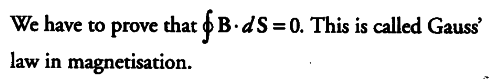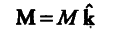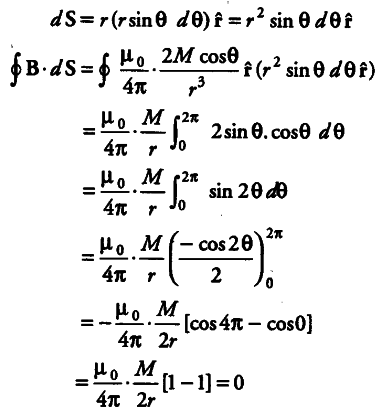# Verify the Gauss’ law for magnetic field

Verify the Gauss’ law for magnetic field of a point dipole of dipole moment M at the origin for the surface which is a sphere of radius R.

Let us draw the figure for given situation,According to the question,
Magnetic moment of dipole at origin O isLet P be a point at distance r from O and OP makes an angle 0 with .Z-axis. Component of M along OP = M cos 0. Now, the magnetic field induction at P due to dipole of moment M cos 0 is
B = { µ }_{ 0 } / 4 \pi . 2M cosθ / {{r}^{3}} r^
From the diagram, r is the radius of sphere with centre at O lying in FZ-plane. Take an elementary area dS of the surface at P. Then,